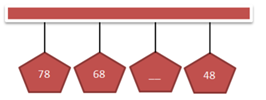Mathematics
Easy

Question

# Which number is missing in the number sequence?## 66    56    58    50Hint:

## The correct answer is: 58

### The given sequence is 78, 68, __, 48We will subtract the second term from first term. We will see if we find a constant, which will help us to write all the terms.78 – 68 = 10Now, we will subtract this value from the second term. We will continue the process till we get the last term. If the term matches the given sequence, then our rule is correct.68 – 10 = 5858 – 10 = 48This matches the fourth term. It means the rule used is to subtract 10.The complete sequence is 78, 68, 58, 48Therefore, the missing term is 58.

For such questions, we should know the basic operations. When the rule used to write the pattern is not mentioned, we have to use all four operations to check the pattern. The operations are addition, subtraction, division, and multiplication.

### Related Questions to study#### With Turito Foundation.#### Get an Expert Advice From Turito.